# Economy's Factors of Production

• Land

• resources that are God-given from nature
• Labor

• work done by human beings
• Capital

• Physical capital: consists of manufactured productive resources like equipment, buildings or tools

• Human capital: skills and knowledge a worker gets from education

• Entrepreneurship

• often referred to as the "4th Factor of Production," they combine the three resources to make innovative profits by taking risk

# Derived Demand

• Definition

• demand from a factor is dericed fomr firm's output.
• Examples

• If the demand for flight increase, then the demand for airline pilots will also increase

• After Hurricane Katrina, the demand for houses increased, thus increasing the demand for construction workers.

• Graph# Total Product vs. Marginal Product

• Total product shows the total quantity of output produced, which will eventually increase at a decreasing rate

• Marginal product of labor will decrease as you hire more workers due to diminishing marginal returns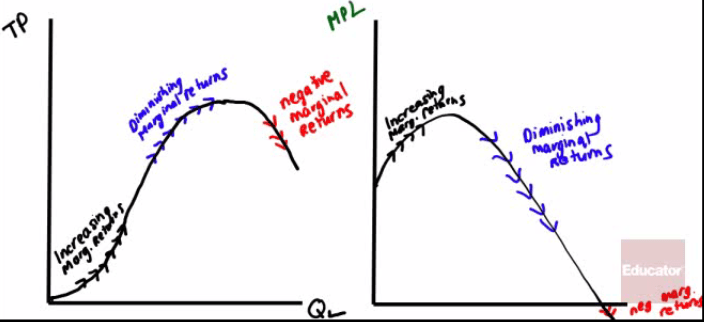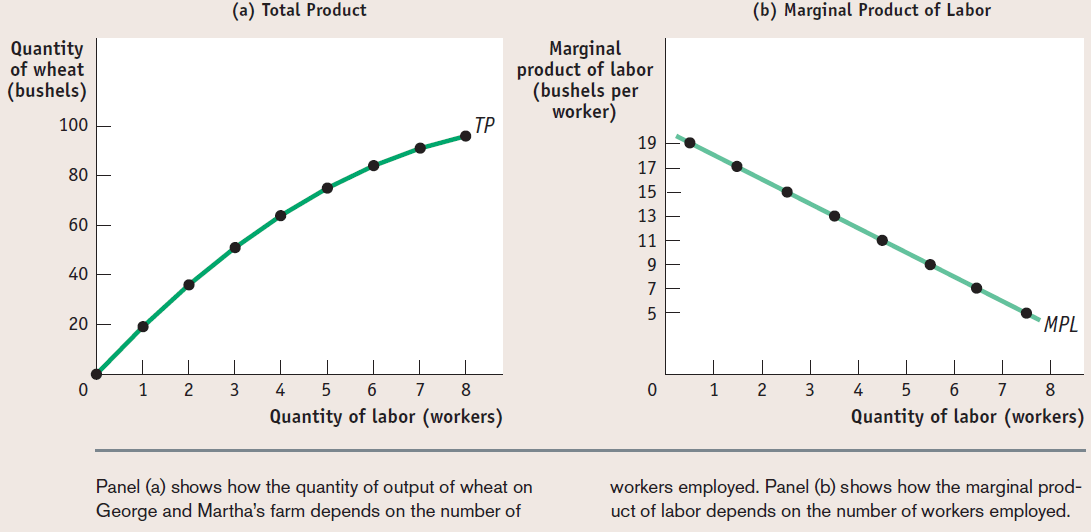# Value of the Marginal Product

• Meaning

• The value of the marginal product of a factor is the value of the additional output generated by employing one more unit of that factor.

VMPL = Value of the Marginal Product of Labor

MRP = Marginal Revenue Product

• Formula

• VMPL = P * MPL

• MRP = P * MPL

• Hiring decision rule

• Hire the extra worker if VMPL >W

• VMPL = W at the profit-maximizing level of employment

• Example

• If the 5th worker produces 7 radios in the one day which sell for \$10 each and his daily wage rate equals \$10, should you hire the worker?

• VMPL = P * MPL = 10 * 7 = 70 < W = 100

• Curve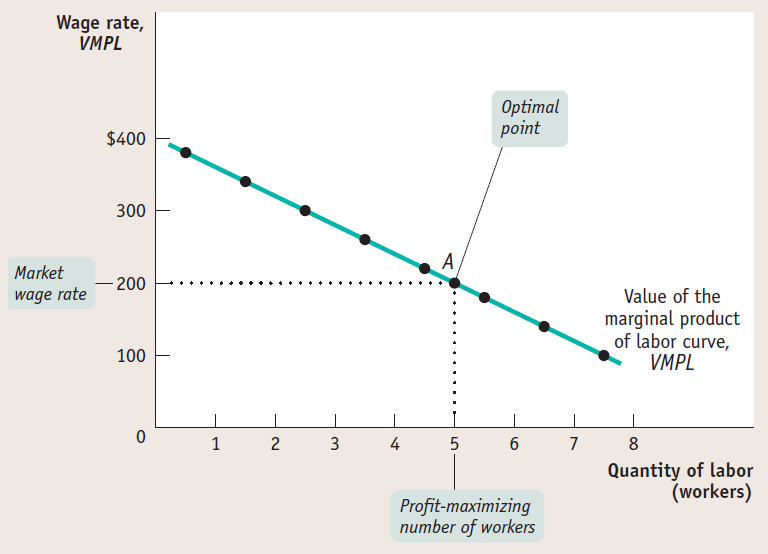• This curve shows how the value of marginal product of labor depends on the number of workers employed.

• It slopes downward because of diminishing returns to labor in production

• To maximize profit, you should choose the level of employment at which the value of the marginal product of labor is equal to the market wage rate

# Shifts of the Factor Demand Curve

• Changes in the price of goods

• If the price of wheat, increases, what happens to the VMPL of wheat?• Change in supply of other factors

• If the workers decrease their productivity due to a loos of land, what happens to the VMPL?• Changes in Technology

• The usual impact of technological process will shift the MPL (and thus VMPL) to the right# Factor Market ExampleAssume that Samantha's Shirt Company sells shirts at \$15 and pays a wage of \$85 a day. Assume labor is the only output.

• Draw a correctly labeled graph of SSC's current supply curve for unskilled labor

• Put QL on the x-axis, and W on the y-axis

• Perfectively competitive labor market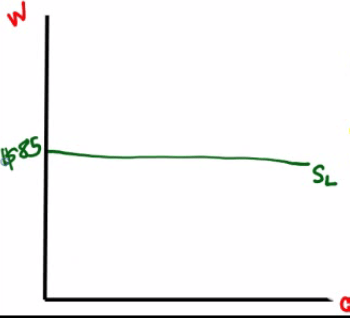• What is SSC's profit maximizing level?

QL Q MPL P MRP/VMPL W
0 0
1 30 30 15 450 85
2 50 20 15 300 85
3 60 10 15 150 85
4 65 5 15 75 85
5 68 3 15 45 85
6 70 2 15 30 85
• When QL = 3, MRP/VMPL > W

• When QL = 4, MRP/VMPL < W

• Answer: Produce 60 shirts and hire 3 workers

• Suppose SSC loses technology that decrease the productivity of its unskilled workers. How will the new technology affect the quantity of unskilled labor SSC hires?

• The MRP decreases, because MPL has decreased.

• The quantity of Labor will decrease

• How will the new technology affect the wage paid to SSC's unskilled workers

• Wage is unaffected because wage is perfectly elastic or constant# Another Factor Market Example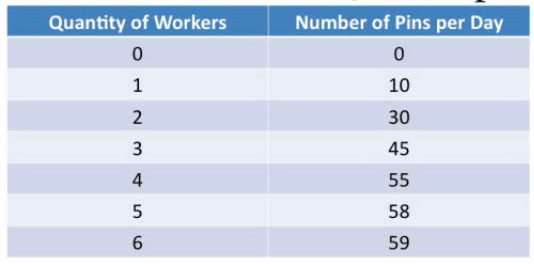Sus & Jib is a profit-maximizing pin-making firm that can sell pins at a price of \$20 each. Sus & Jib can hire workers at a market wage of \$120 per day per worker

• In what market structure does this company sell its products?

• perfect competition because price is the same and no differentiation.
• In what market structure do the laborers work in?

• perfect competition because wage rate is constant
• Calculate the value of the marginal product of labor of the third worker

• MRP = (45 - 30) * 30 = 15 * 20 = 300
• What is the profit-maximizing output level?

QL Q MPL P MRP/VMPL W
0 0
1 10 10 20 200 120
2 30 20 20 400 120
3 45 15 20 300 120
4 55 10 20 200 120
5 58 3 20 60 120
6 59 1 20 30 120
• Profit-maximizing output level is 55-58 pins with 4-5 workers